# SlopePage 1

#### WATCH ALL SLIDES

Slide 1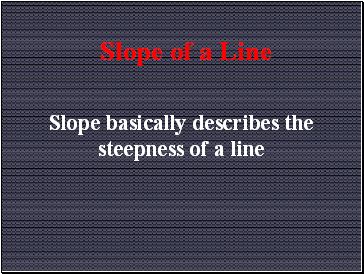## Slope of a Line

Slope basically describes the steepness of a line

Slide 2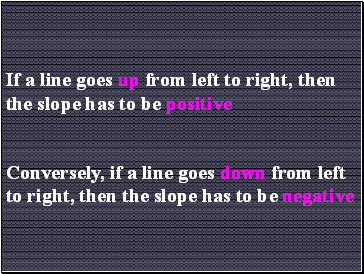If a line goes up from left to right, then the slope has to be positive

Conversely, if a line goes down from left to right, then the slope has to be negative

Slide 3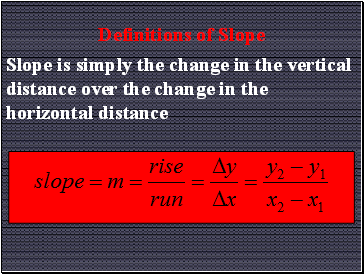## Definitions of Slope

Slope is simply the change in the vertical distance over the change in the horizontal distance

Slide 4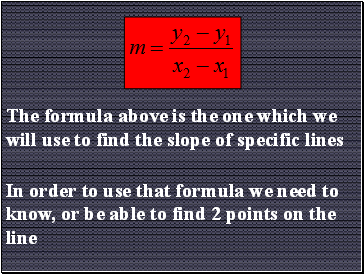The formula above is the one which we will use to find the slope of specific lines

In order to use that formula we need to know, or be able to find 2 points on the line

Slide 5If a line is in the form Ax + By = C, we can use the following formula to find the slope:

Slide 6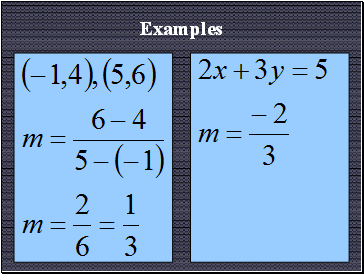Examples

Slide 7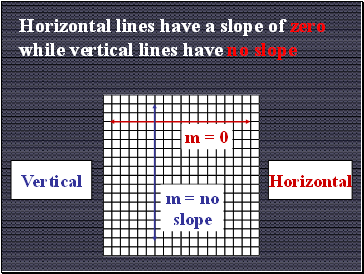Horizontal lines have a slope of zero while vertical lines have no slope

Horizontal

Vertical

m = 0

m = no slope